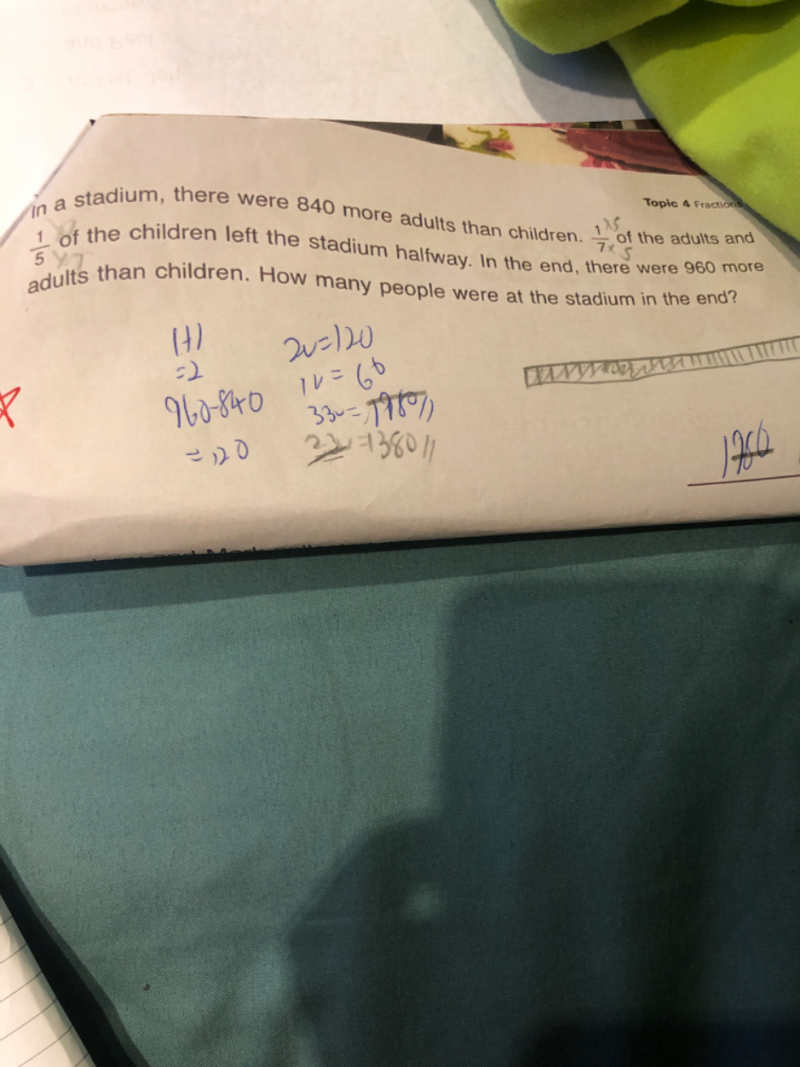# QuestionPls assist

At first                                 u + 840                         u

Left  stadium                  (1/7)(u+840)               (1/5)u

At the end                       (6/7)(u+840)              (4/5)u

Given at the end, adults is 960 more than children,

(6/7)(u+840) –  (4/5)u   = 960    (x 35 on both sides)

30u + 25200 – 28u = 33600

2u = 8400

u = 4200

At first adults = 4200 + 840 = 5040 ,   after 1/7 left,  adults = (6/7) x 5040 = 4320

At first children = 4200, after 1/5 left, children = (4/5) x 4200 = 3360

Hence at the end, number of people = 4320+3360 = 7680

0 Replies 1 Like